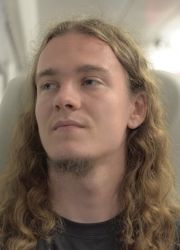Marcel Serina

Contact

Department of Physics
University of Basel
Klingelbergstrasse 82
CH-4056 Basel, Switzerland
 office: 4.8 email: view address tel: +41 (0)61 207 37 46Research interests

• Majorana fermions in condensed matter systems
• Qubits in quantum dots
• DMRG-based analysis of interacting systems

Publications

Show all abstracts.

 1. Free Energy Analysis of Spin Models on Hyperbolic Lattice Geometries Marcel Serina, Jozef Genzor, Yoju Lee, and Andrej Gendiar. arXiv:1511.08358; Phys. Rev. E 93, 042123 (2016). We investigate relations between spatial properties of the free energy and the radius of Gaussian curvature of the underlying curved lattice geometries. For this purpose we derive recurrence relations for the analysis of the free energy normalized per lattice site of various multistate spin models in the thermal equilibrium on distinct non-Euclidean surface lattices of the infinite sizes. Whereas the free energy is calculated numerically by means of the corner transfer matrix renormalization group algorithm, the radius of curvature has an analytic expression. Two tasks are considered in this work. First, we search for such a lattice geometry, which minimizes the free energy per site. We conjecture that the only Euclidean flat geometry results in the minimal free energy per site regardless of the spin model. Second, the relations among the free energy, the radius of curvature, and the phase transition temperatures are analyzed. We found out that both the free energy and the phase transition temperature inherit the structure of the lattice geometry and asymptotically approach the profile of the Gaussian radius of curvature. This achievement opens new perspectives in the AdS-CFT correspondence theories. 2. Long-Range Interaction between Charge and Spin Qubits in Quantum Dots Marcel Serina, Christoph Kloeffel, and Daniel Loss. arXiv:1601.03564; Phys. Rev. B 95, 245422 (2017). We analyze and give estimates for the long-distance coupling via floating metallic gates between different types of spin qubits in quantum dots made of different commonly used materials. In particular, we consider the hybrid, the singlet-triplet, and the spin-1/2 qubits, and the pairwise coupling between each type of these qubits with another hybrid qubit in GaAs, InAs, Si, and Si0.9Ge0.1. We show that hybrid qubits can be capacitively coupled strongly enough to implement two-qubit gates, as long as the dimensions of the dots and their distance from the metallic gates are small enough. Thus, hybrid qubits are good candidates for scalable implementations of quantum computing in semiconducting nanostructures.